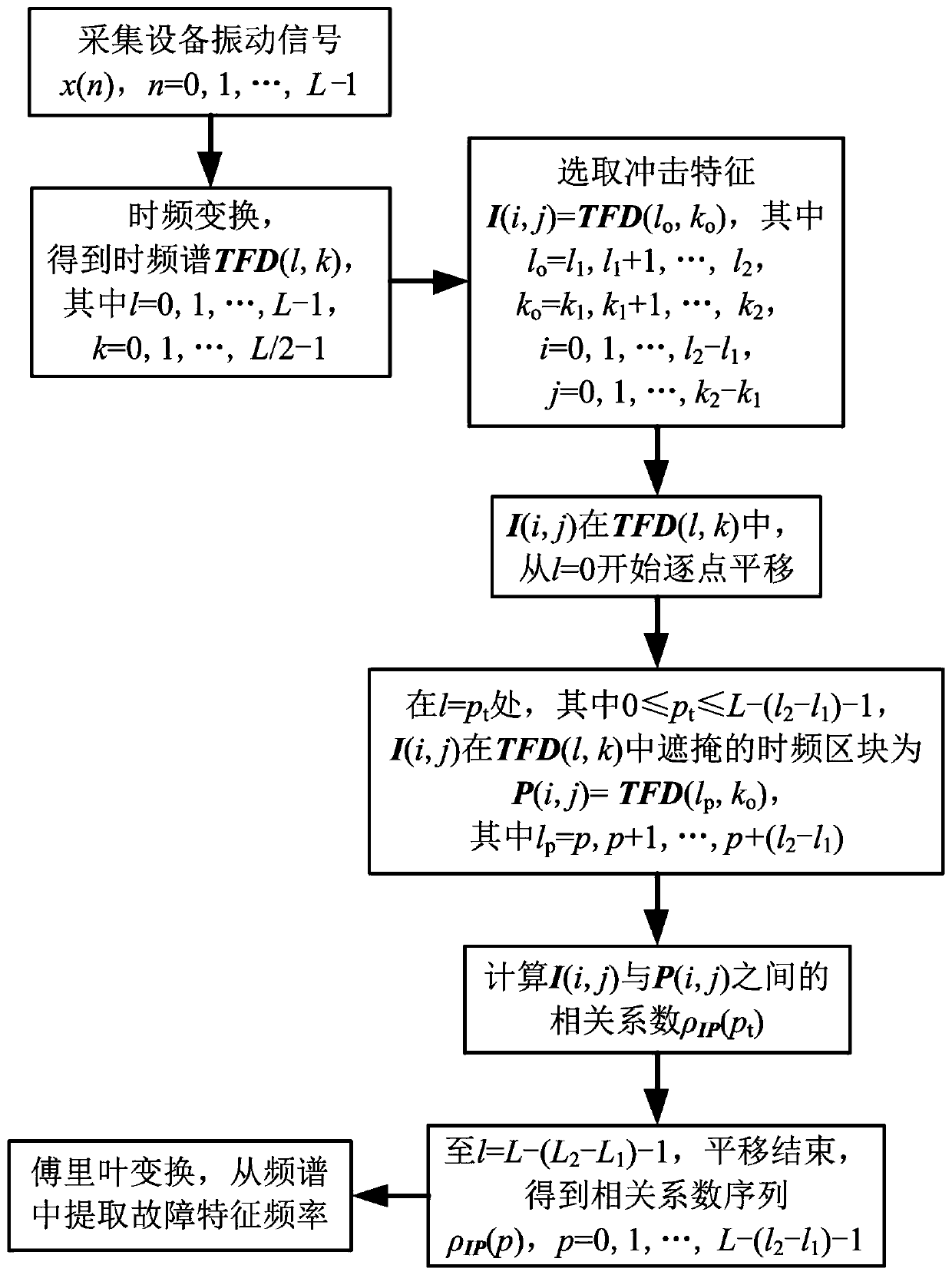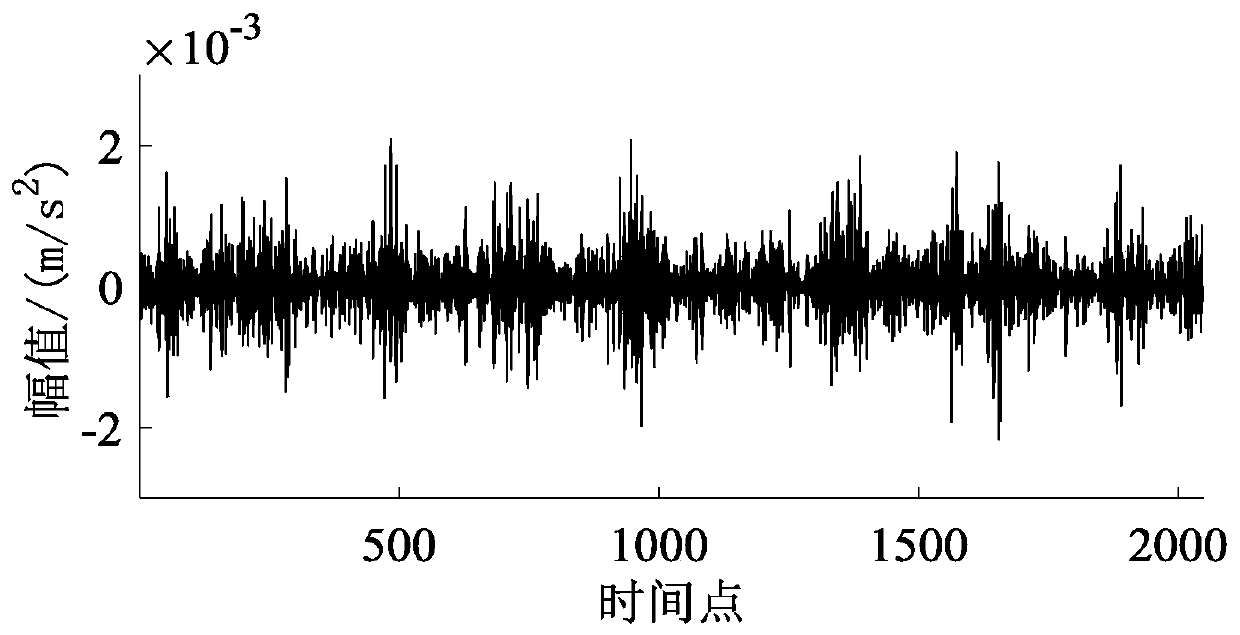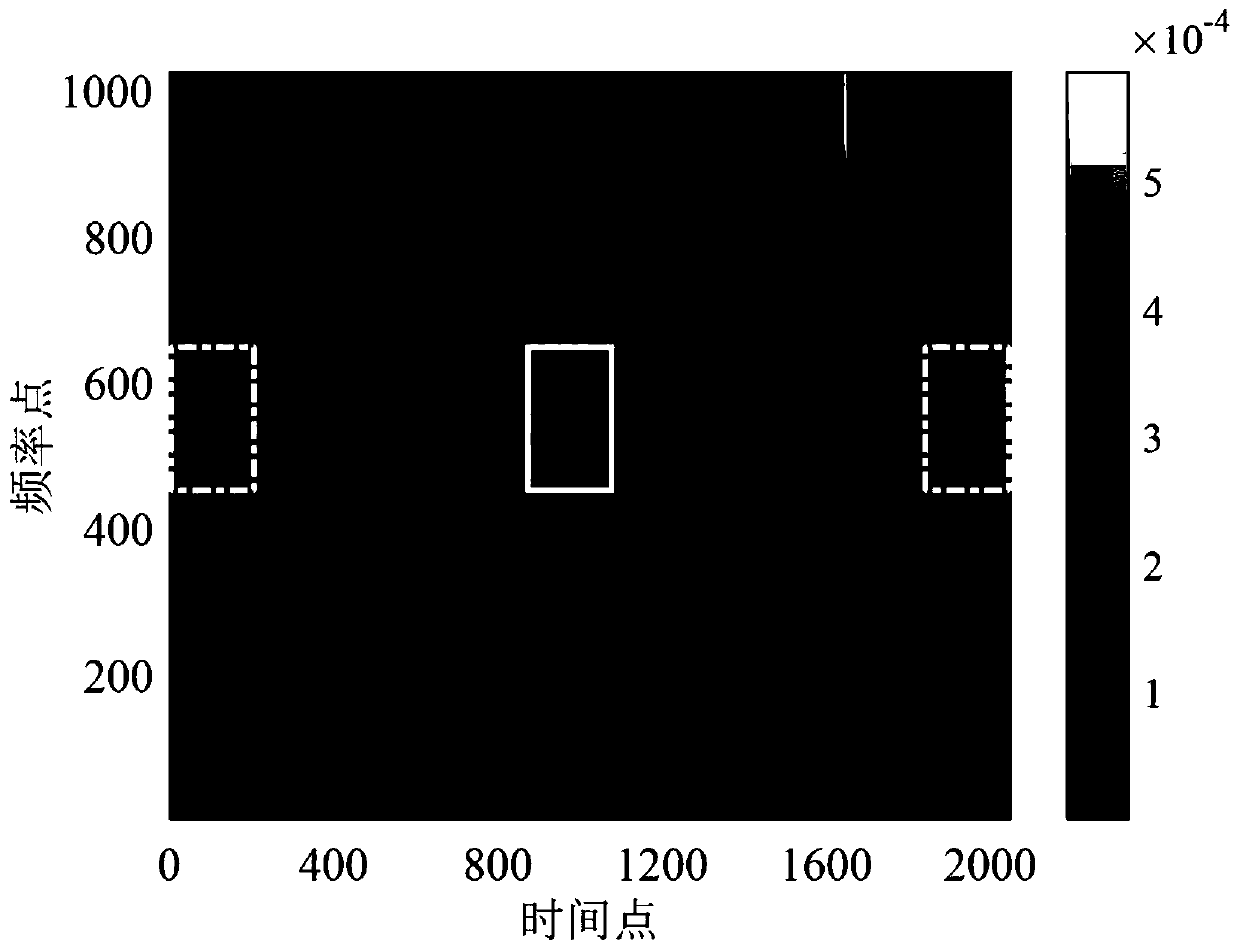# A rotary machine fault feature extraction method based on time-frequency spectrum correlation analysis

## A correlation analysis and rotating machinery technology, applied in the testing of mechanical components, computer components, machine/structural components, etc., can solve the problem of inability to reduce noise, difficulty in effectively extracting fault characteristic frequencies, and unintuitive regularity of impact characteristics Display and other problems to achieve the effect of removing noise and other interference signals and removing influence

Active Publication Date: 2019-06-28
ZHEJIANG COLLEGE OF ZHEJIANG UNIV OF TECHOLOGY
3 Cites 1 Cited by

## AI-Extracted Technical Summary

### Problems solved by technology

However, the time-frequency analysis method of the vibration signal itself cannot reduce the noise. For the fault vibration signal of the rotating mechanical equipment with a low signal-to-noise ratio, only a few shock features with high energy are often highlighted in...
the structure of the environmentally friendly knitted fabric provided by the present invention; figure 2 Flow chart of the yarn wrapping machine for environmentally friendly knitted fabrics and storage devices; image 3 Is the parameter map of the yarn covering machine
View more

## Abstract

The rotary machine fault feature extraction method based on time-frequency spectrum correlation analysis comprises the following steps: firstly, collecting a group of vibration signals on rotary machine equipment, and carrying out time-frequency transformation on the vibration signals to obtain a time-frequency spectrum; secondly, selecting an impact feature in the time-frequency spectrum, enabling the impact feature to perform point-by-point translation along a time axis from the initial time of the time-frequency spectrum, continuously masking a time-frequency block in the time-frequency spectrum, calculating a correlation coefficient between the impact feature and the masked time-frequency block, and obtaining a correlation coefficient sequence after translation is finished; finally, performing Fourier transform on the correlation coefficient sequence, and extracting the fault characteristic frequency from the frequency spectrum. The obtained correlation coefficient sequence curvecan visually display the change rule of the impact characteristic in the vibration signal of the rotary mechanical equipment, and the influence of noise and other interference signals is greatly removed; and the frequency spectrum of the correlation coefficient sequence can effectively and conveniently extract the fault characteristic frequency for fault diagnosis of the rotary mechanical equipment.

Application Domain

Technology Topic

## Image

•••## Examples

• Experimental program(1)

### Example Embodiment

 The present invention will be further described below with reference to the drawings and embodiments.
 Reference figure 1 , The method for extracting fault features of rotating machinery based on time-frequency correlation analysis includes the following steps:
 (1) Collect a set of vibration acceleration signals x(n) of length L on the rotating machinery and equipment, where n=0,1,...,L-1;
 (2) Perform time-frequency transformation on the signal x(n) to obtain the time-frequency spectrum TFD(l,k), where time l=0,1,...,L-1, frequency k=0,1,...,L/2 -1;
 (3) Select two time-frequency points (l, k) in the time-frequency spectrum TFD(l,k) 1 ,k 1 ), (l 2 ,k 2 ) To select an impact feature I(i,j), where time i=0,1,...,l 2 -l 1 , Frequency j=0,1,...,k 2 -k 1; The time-frequency block where the impact feature I(i,j) is located is TFD(l o ,k o ), that is, I(i,j)=TFD(l o ,k o ), where time l o = L 1 ,l 1 +1,…,l 2 , Frequency k o =k 1 ,k 1 +1,...,k 2;
 (4) In the time spectrum TFD(l,k), keep the frequency band [k 1 ,k 1 +1,...,k 2 ] Unchanged, let the impact feature I(i,j) start from the time point l=0 and move along the time axis point by point;
 (5) At time point l=p t Where 0≤p t ≤L-(l 2 -l 1 )-1, the time-frequency block concealed by the impact feature I(i,j) in the time-frequency spectrum TFD(l,k) is P(i,j)=TFD(l p ,k o ), where time l p = P t ,p t +1,...,p t +(l 2 -l 1 );
 (6) Calculate the correlation coefficient ρ between the impact feature I(i,j) and the masked time-frequency block P(i,j) IP (p t ), the calculation formula is:

 among them Is the mean value of impact feature I(i,j), the calculation formula is:

 To conceal the average value of the time-frequency block P(i,j), the calculation formula is:

 (7) The impact feature I(i,j) is translated to the time point l=L-(l 2 -l 1 )-1, the translation ends, and the impact features I(i,j) and [L-(l 2 -l 1 )] a sequence of correlation coefficients between time-frequency blocks ρ IP (p), where p=0,1,...,L-(l 2 -l 1 )-1;
 (8) For the correlation coefficient sequence ρ IP (p) Perform Fourier transform to extract the fault characteristic frequency from the frequency spectrum.
 The time-frequency transformation algorithm in the step (2) includes S transformation, and the calculation formula is:

 Where S(l,k) is the element value of the S transform spectrum at the time-frequency point (l,k), L is the length of the signal x(n), and X(m) is the Fourier transform of the signal x(n) , The calculation formula is:

 The time spectrum TFD(l,k) is equal to the absolute value of the S transform spectrum, that is, TFD(l,k)=|S(l,k)|.
 The above invention is applied to the fault vibration signal processing of the gearbox. The gearbox used is a one-stage parallel shaft gearbox, the speed increasing ratio is 2.45, the input shaft speed is 550r/min, the output shaft speed is 1347r/min, the torque load of the output shaft is 3N·m, and the internal pinion is one wheel Partial fracture damage occurs to the tooth. Through calculation, it can be known that the characteristic frequency of the pinion gear is 22.45 Hz. The sampling frequency of the vibration signal of the gearbox is set to 5.12 kHz. The steps of extracting fault features using the present invention are as follows.
 (1) On the gearbox used, use a vibration sensor to collect a set of vibration acceleration signals x(n) with a length of L=2048, where n=0,1,...,2047, such as figure 2 Shown
 (2) Perform S transform on the group of vibration signals x(n) to obtain the time spectrum TFD(l,k), where time l=0,1,...,2047, frequency k=0,1,...,1023, such as image 3 Shown
 (3) Select two time-frequency points (867,460), (1068,664) in the time-frequency spectrum TFD(l,k) to select an impact feature I(i,j), where i=1, 2,..., 202, j=1,2,...,205; such as image 3 As shown by the solid-line framed area in the middle, the time-frequency block where the impact feature I(i,j) is located is TFD(l o ,k o ), that is, I(i,j)=TFD(l o ,k o ), where l o =867,868,...,1068, k o =460,461,...,664.
 (4) In the time-frequency spectrum TFD(l,k), keep the frequency band [460,461,...,664] unchanged, make the impact feature I(i,j) start from the time point l=0, and move along the time axis point by point .
 (5) At time point l=p t Where 0≤p t ≤1846, the time-frequency block covered by the impact feature I(i,j) in the time-frequency spectrum TFD(l,k) is P(i,j)=TFD(l p ,k o ), where time l p = P t ,p t +1,...,p t +201, and at time l=0, the masked time-frequency block is as image 3 The left-most dot-dash line frame selection area is shown.
 (6) Calculate the correlation coefficient ρ between the impact feature I(i,j) and the masked time-frequency block P(i,j) IP (p t ), the calculation formula is:

 among them versus They are the mean values ​​of the impact feature I(i,j) and the masked time-frequency block P(i,j). The calculation formulas are:


 (7) After the impact feature I(i,j) is translated to the time point l=1846, the translation ends. At this time, the time-frequency block covered is as image 3 As shown in the dotted line frame selection area on the far right of, the correlation coefficient sequence ρ between the impact feature I(i,j) and 1847 masked time-frequency blocks is finally obtained IP (p), where p=0,1,...,1846, the curve is as Figure 4 Shown.
 (8) For the correlation coefficient sequence ρ IP (p) Carry out fast Fourier transform, the frequency spectrum obtained is as Figure 5 As shown, the fault characteristic frequency extracted from it is 22.18 Hz, which is consistent with the fault characteristic frequency of the pinion, indicating that the pinion in the gearbox is locally damaged.
 The content described in the embodiments of this specification is merely a list of the realization forms of the inventive concept, and the protection scope of the present invention should not be regarded as limited to the specific forms stated in the embodiments. The protection scope of the present invention also extends to the technology in the field. People can think of equivalent technical means based on the concept of the present invention.
the structure of the environmentally friendly knitted fabric provided by the present invention; figure 2 Flow chart of the yarn wrapping machine for environmentally friendly knitted fabrics and storage devices; image 3 Is the parameter map of the yarn covering machine

## PUM## Description & Claims & Application Information

We can also present the details of the Description, Claims and Application information to help users get a comprehensive understanding of the technical details of the patent, such as background art, summary of invention, brief description of drawings, description of embodiments, and other original content. On the other hand, users can also determine the specific scope of protection of the technology through the list of claims; as well as understand the changes in the life cycle of the technology with the presentation of the patent timeline. Login to view more.
the structure of the environmentally friendly knitted fabric provided by the present invention; figure 2 Flow chart of the yarn wrapping machine for environmentally friendly knitted fabrics and storage devices; image 3 Is the parameter map of the yarn covering machine

## Method and device for detecting lip state

Owner:BEIJING SOGOU TECHNOLOGY DEVELOPMENT CO LTD

## Mass spectrometer and mass spectrometry method

PendingUS20220216042A1Remove influenceReduce number of repetitionSamples introduction/extractionParticle spectrometer methodsComputational physicsEngineering

## Device and method for processing photoacoustic signal

ActiveUS20140257079A1Remove influenceUltrasonic/sonic/infrasonic diagnosticsProcessing detected response signalPhysicsPhotoacoustic imaging in biomedicine
Owner:FUJIFILM CORP

## Hybrid altimeter for measuring vertical velocity

ActiveUS20190064199A1Remove influenceSpeed measurement using accelerationNavigation by speed/acceleration measurementsReal-time computingPressure sensor
Owner:OTIS ELEVATOR CO

## Data processing system and program for chromatograph mass spectrometer

ActiveUS20170067865A1Reduce laborRemove influenceParticle separator tubesComponent separationPhysicsSignal intensity

## Classification and recommendation of technical efficacy words

• Remove influence

## User equipment for simultaneously transmitting signals to which different wireless communication systems are applied through a plurality of frequency bands

Owner:LG ELECTRONICS INC

## Coordinate calculating apparatus and coordinate calculating program

Owner:NINTENDO CO LTD

## Apparatus and Method for Detecting Frame Boundary in Wireless Communication System

Owner:WIRELESS ALLIANCE LLC

## Method of Manufacturing Solar Battery

Owner:SANYO ELECTRIC CO LTD

## Internal Combustion Engine Knock Determination Device and Knock Determination Method

Owner:TOYOTA JIDOSHA KK +2
Who we serve
• R&D Engineer
• R&D Manager
• IP Professional
Why Eureka